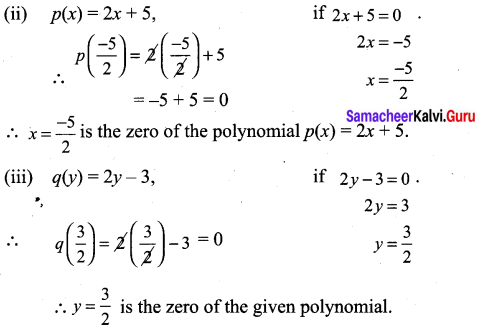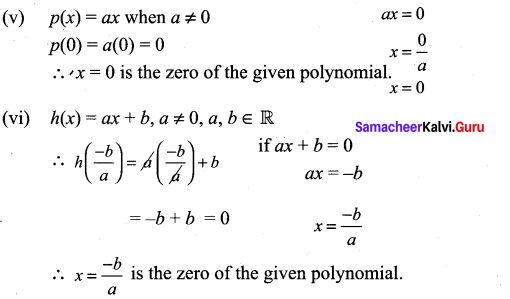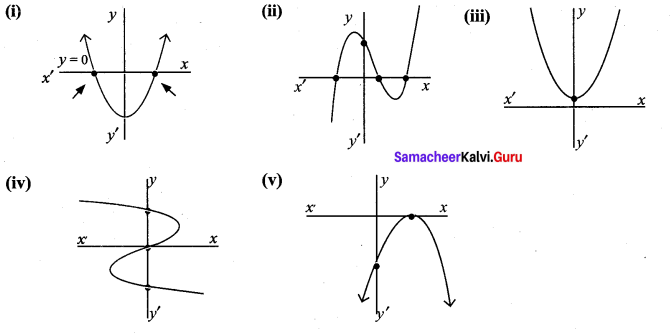# Samacheer Kalvi 9th Maths Solutions Chapter 3 Algebra Ex 3.2

## Tamilnadu Samacheer Kalvi 9th Maths Solutions Chapter 3 Algebra Ex 3.2

9th Maths Algebra Exercise 3.2 Question 1.
Find the value of the polynomial f(y) = 6y – 3y2 + 3 at (i) y = 1 (ii) y = -1 (iii) y = 0
Solution:
(i) At y = 1,
f(1) = 6(1) – 3(1)2 + 3 = 6 – 3 + 3 = 6
(ii) At y = -1,
f(-1) = 6(-1) – 3(-1)2 + 3 = -6 – 3 + 3 = -6
(iii) At y = 0,
f(0) = 6(0) – 3(0)2 + 3 = 0 – 0 + 3 = 3

9th Maths Exercise 3.2 Question 2.
If f(x) = x2 – $$2 \sqrt{2} x$$ + 1, find p ($$2 \sqrt{2}$$)
Solution:
p($$2 \sqrt{2}$$) = ($$2 \sqrt{2}$$)2 – $$2 \sqrt{2}$$($$2 \sqrt{2}$$) + 1
= 4 × 2 – 4 × 2 + 1
= 8 – 8 + 1 = 1

Ex 3.2 Class 9 Algebra Question 3.
Find the zeros of the polynomial in each of the following :
(i) p(x) = x – 3
(ii) p(x) = 2x + 5
(iii) q(y) = 2y – 3,
(iv) f(z) = 8z
(v) p(x) = ax where a ≠ 0,
(vi) h(x) = ax + b, a ≠ 0, a, b ∈ R
Solution:
(i) x = 3.
p( 3) = 3 – 3 = 0
∴ The zero of the polynomial is x = 3.(iv) f(z) = 8z,
If 8z = 0
z = $$\frac{0}{8}$$ = 0
f(0) = 8(0) = 0
∴ z = 0 is the zero of the given polynomial.Class 9 Maths Exercise 3.2 Solutions Question 4.
Find the roots of the polynomial equations.
(i) 5x – 6 = 0
(ii) x + 3 = 0
(iii) 10x + 9 = 0
(iv) 9x – 4 = 0
Solution:
(i) 5x – 6 = 0
5x = 6
∴ x = $$\frac{6}{5}$$

(ii) x + 3 = 0
∴ x = -3

(iii) 10x + 9 = 0
10x = -9
∴ x = $$\frac{-9}{10}$$

(iv) 9x – 4 = 0
9x = 4
∴ x = $$\frac{4}{9}$$

Verify whether the following are zeros of the polynomial indicated against them, or not.
(i) p(x) = 2x – 1, x = $$\frac{1}{2}$$
(ii) p(x) = x3 – 1, x = 1,
(iii) p(x) = ax + b, x = $$\frac{-b}{a}$$
(iv) p(x) = (x + 3) (x – 4), x = 4, x = -3
Solution:
(i) p(x) = 2x – 1, x = $$\frac{1}{2}$$
p($$\frac{1}{2}$$) = 2($$\frac{1}{2}$$) – 1 = 1 – 1 = 0
∴ x = $$\frac{1}{2}$$ is the zero of the given polynomial.

(ii) p(x) = x3 – 1, x = 1
p(1) = 13 – 1 = 1 – 1 = 0
∴ x = 1 is the zero of the given polynomial

(iii) p(x) = ax + b, x = $$\frac{-b}{a}$$
p($$\frac{-b}{a}$$) = a($$\frac{-b}{a}$$) + b
= -b + b = 0
∴ x = $$\frac{-b}{a}$$ is the zero of the given polynomial.

(iv) p(x) = (x + 3) (x – 4), x = 4, x = -3
p(-3) = (-3 + 3) (-3 – 4) = 0(-7) = 0
p(4) = (4 + 3) (4 – 4) = 7(0) = 0
∴ x = -3, x = 4 are the zeros of the given polynomial.

Samacheer Kalvi 9th Standard Maths Question 6.
Find the number of zeros of the following polynomial represented by their graphs.Solution:
(i) The curve cuts the x-axis at two points. ∴ The equation has 2 zeros.
(ii) Since the curve cuts the x-axis at 3 different points. The number of zeros of the given curve is three.
(iii) Since the curve doesn’t cut the x axis. The number of zeros of the given curve is zero.
(iv) The curve cut the x-axis at one point. ∴ The equation has one zero.
(v) The curve cut the x axis at one point. ∴ The equation has one zero.